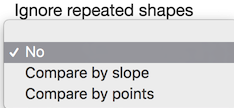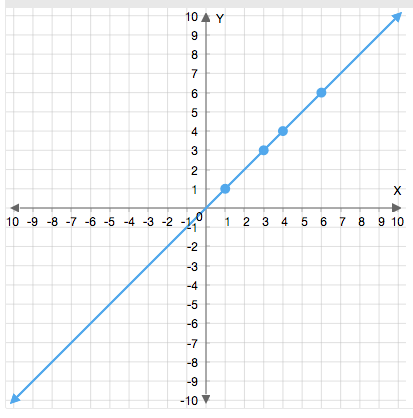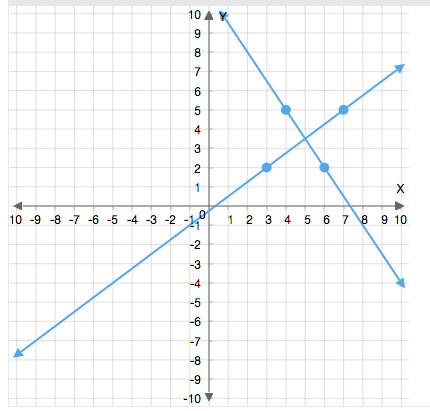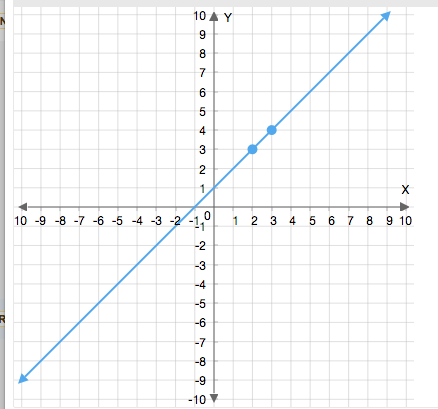# Using the Repeated Shapes Option in Graphing

In the graph plotting question, it is easy for authors or students to plot duplicate shapes on top of each other without realising. It is impossible to tell if there are duplicate shapes from a pure visual inspection. Duplicate shapes can cause validation to fail if the Ignore repeated Shapes option is not switched on.

To avoid this issue, authors can use the Ignore Repeated Shapes flag. This can be found in Validation.Figure 1: Ignore repeated shapes drop down menu in the Question Editor.

The possible options are:

 Value Result No Repeated shapes are not ignored. Compare by slope Repeated shapes are ignored even if the points are not equal, e.g. the two lines here would be considered equivalent because the slopes of the line are equal.These two lines would not be considered equivalent:Parallel lines are also not considered as equivalent. Compare by points Repeated shapes are ignored only if the points are equal. e.g. the two lines here would be considered equivalent because the points are equal.These two lines would not be considered equivalent: